#Function Repository Resource:

# WhiteningTransform

Transform data such that its covariance matrix is the identity matrix

Contributed by: Sander Huisman
 ResourceFunction["WhiteningTransform"][data] whitens data such that its covariance matrix is the identity matrix.

## Details and Options

The whitening transform is sometimes called the sphering transformation.
data should be a list of vectors with equal length.
The number of data points should be more than the length of each vector, in other words, for N-dimensional data, at least N+1 data points are needed.
Using principal component analysis, data is transformed such that the covariance matrix of ResourceFunction["WhiteningTransform"][data] is the identity matrix.
ResourceFunction["WhiteningTransform"] takes a method option, with settings Automatic (default) and "SVD".
The Method "SVD" is generally more numerically stable, as it computes a singular value decomposition. So the covariance matrix is closer to an identity matrix. This is, in general, slower as compared to the default algorithm. Note that the different methods can cause the values to be multiplied -1 for each dimension: principal axes are only defined up to a constant.

## Examples

### Basic Examples (3)

Show some original data:

 In:=Out=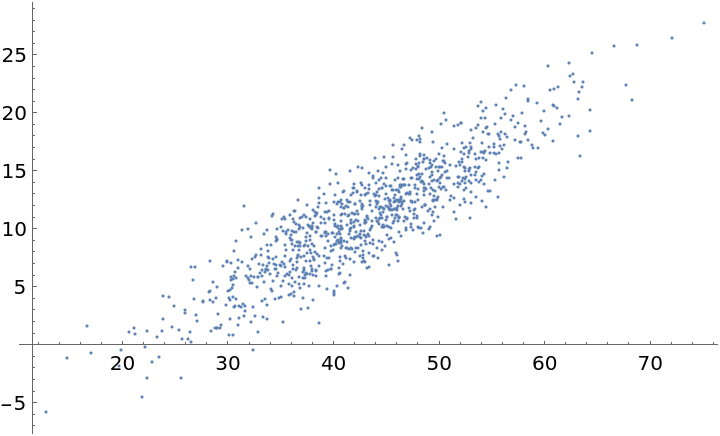Apply the whitening transform and show the result:

 In:=Out=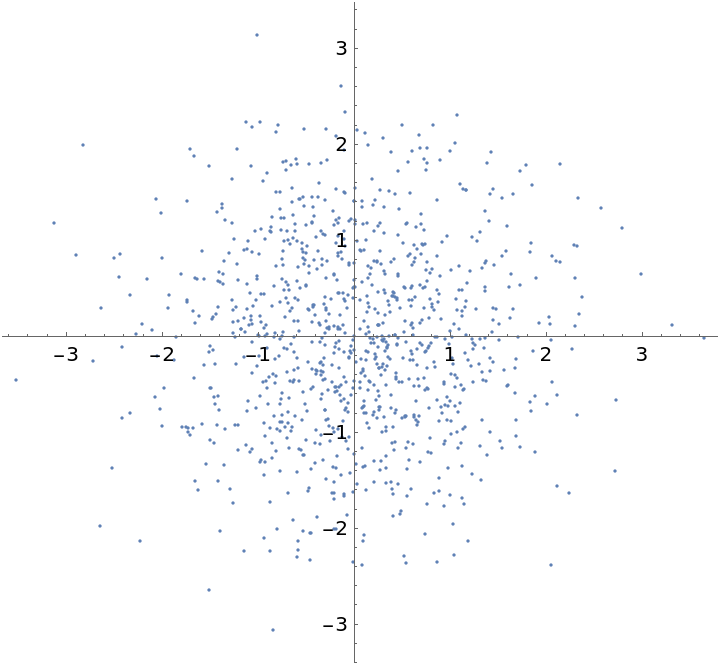Verify that the covariance matrix is an identity matrix (up to small numerical errors):

 In:=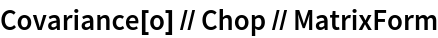Out=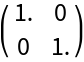### Scope (2)

Data can also be in higher dimensions:

 In:=Out=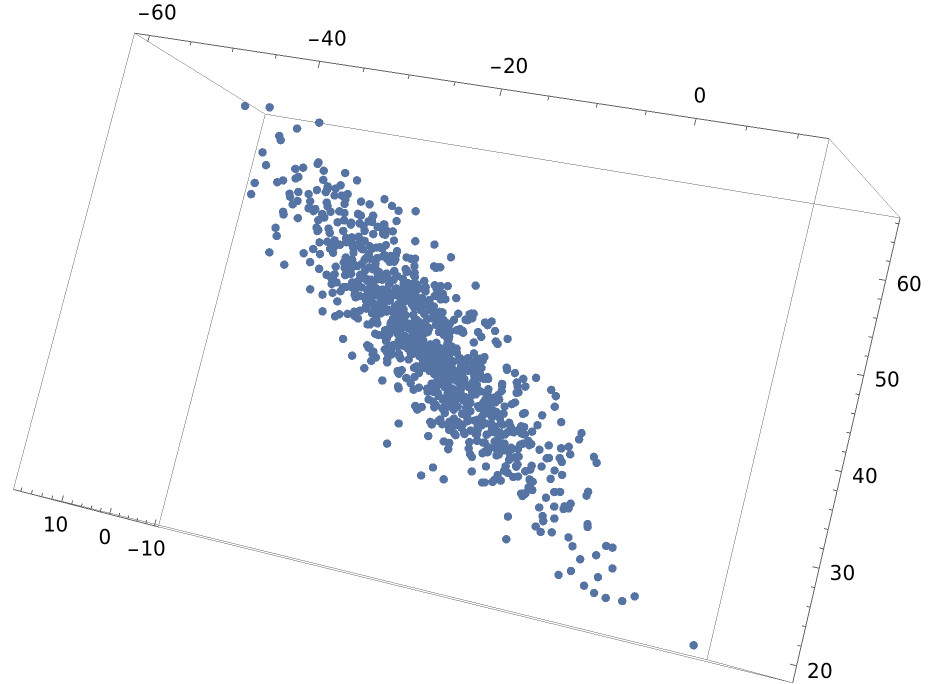Apply the transform:

 In:=Out=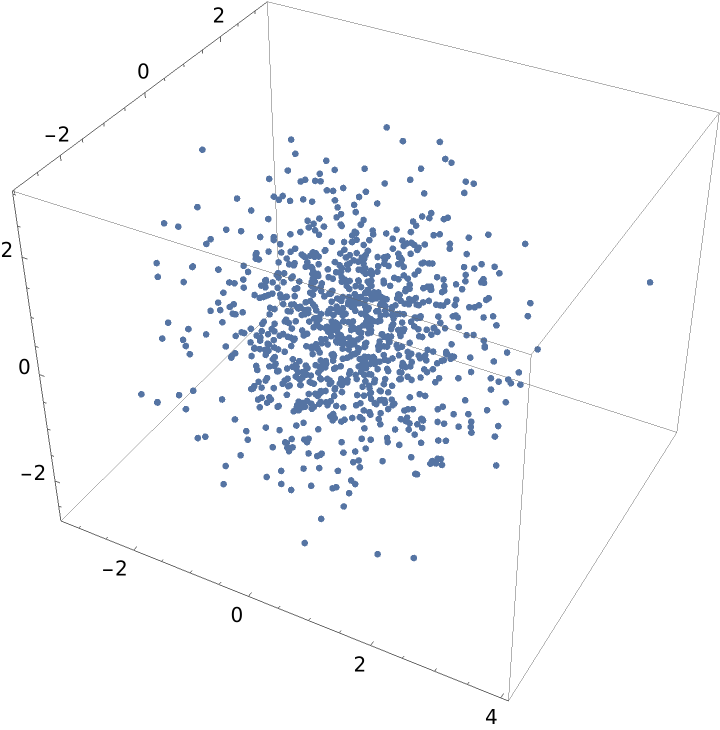Verify that the covariance matrix is the identity matrix up to numerical error:

 In:=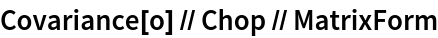Out=Use the default method and the "SVD" method and check how far the covariance matrices deviate from the identity matrix:

 In:=Out=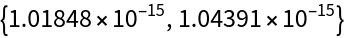### Neat Examples (4)

Create some 6 dimensional correlated data and visualize it:

 In:=Out=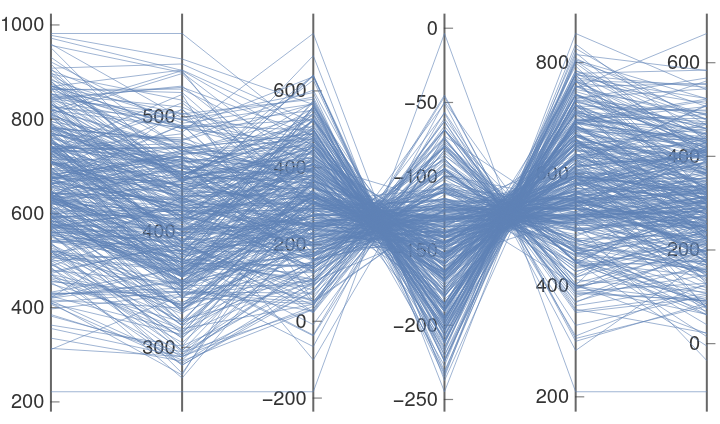Show the plots of 2D projections:

 In:=Out=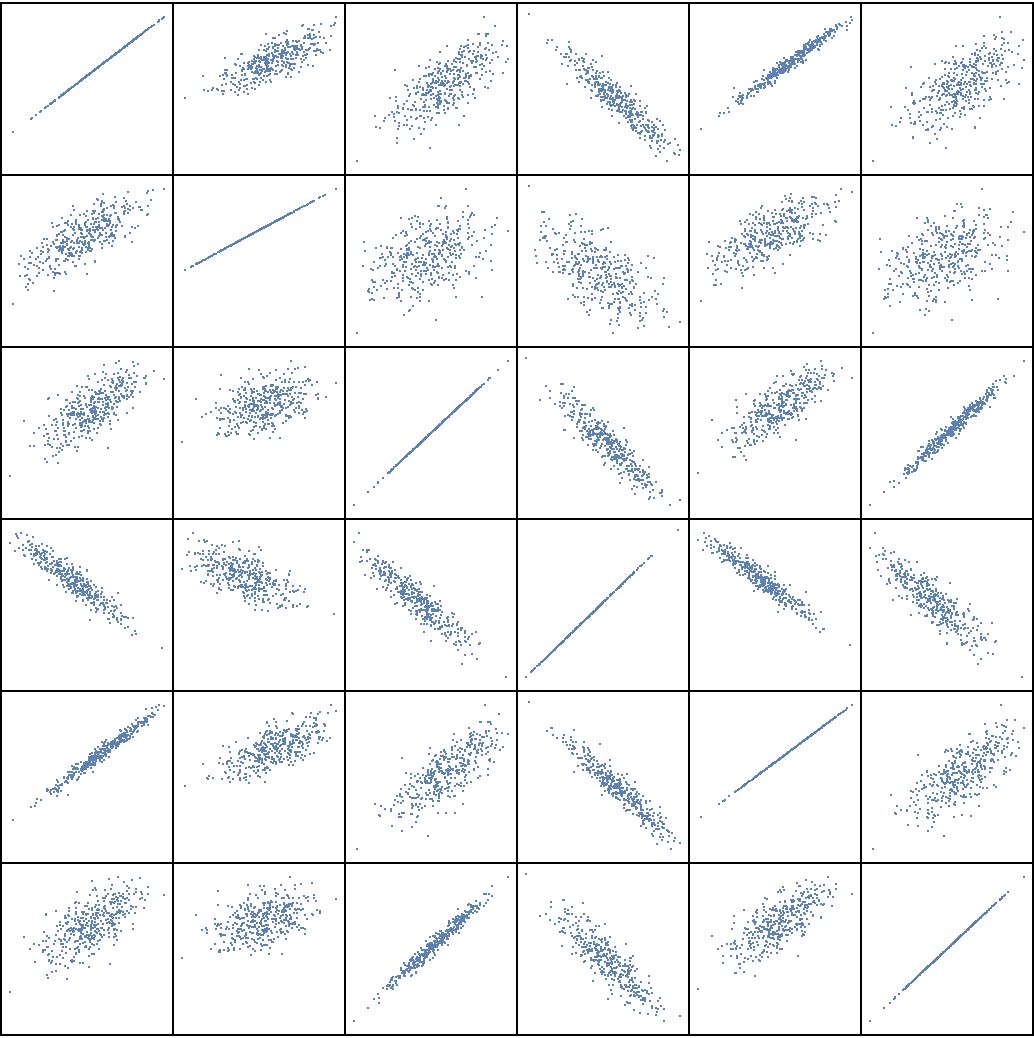Whiten the data and visualize the output to verify that all off-diagonal plots are "sphered" and the diagonals are on a line:

 In:=Out=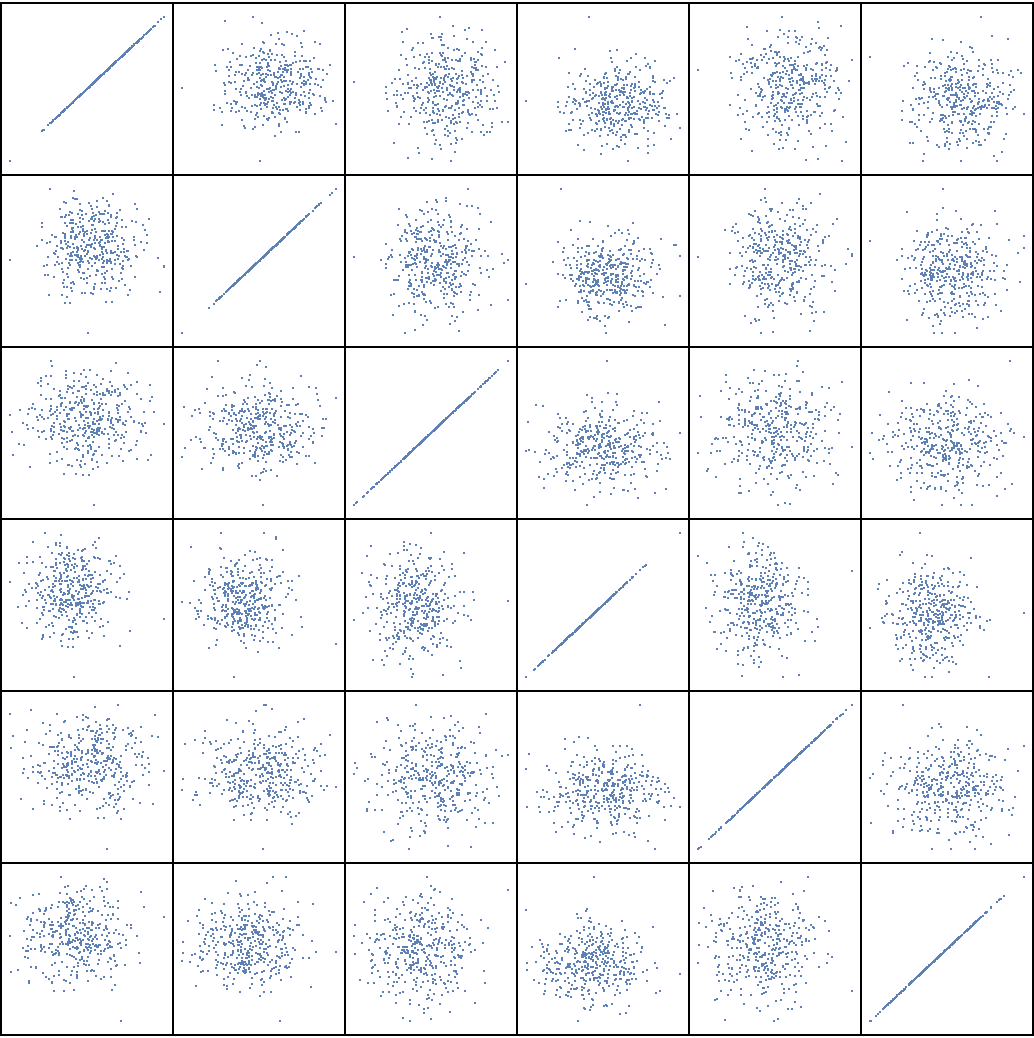Verify that the covariance matrix is the identity matrix:

 In:=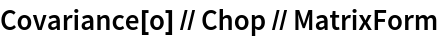Out=